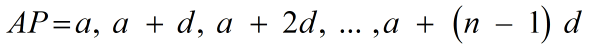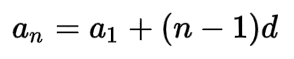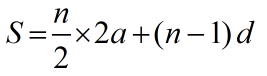Request a Tool

Arithmetic Progression Calculator

Arithmetic Progression Calculator use to calculate arithmetic sequence, nth term value and sum of all terms until 3rd.

Output

Arithmetic Sequence
0

Formula• AP = Arithmetic Progression
• a = The first term in the sequence
• n = The final term in the sequence
• d = The common difference between terms

Defination / Uses

The difference between consecutive terms in an arithmetic progression or arithmetic sequence is constant. An arithmetic series is composed of the sum of a finite arithmetic progression.

Nth term value
0

Formula• an = The nᵗʰ term in the sequence
• a1 = The first term in the sequence
• n = The final term in the sequence
• d = The common difference between terms

Defination / Uses

An arithmetic progression is a series in which each term is a specific number greater than the one before it. 2n + 1 is the nth term in this sequence. a + (n - 1)d is the nth term of an arithmetic progression with first term a and common difference d.

Output

Sum of all terms until 3rd
0

Formula• S = Sum of arithmetic progression
• a = The first term in the sequence
• n = The final term in the sequence
• d = The common difference between terms

Defination / Uses

The total of the terms in an arithmetic sequence is called an arithmetic series. The initial and last terms of an arithmetic series can be used to calculate the nth partial sum.

The whole step-by-step calculation for obtaining the nth term and the nth partial sum of an arithmetic progression where there are 5 terms in the arithmetic progression, the first term is 5, and the common difference is 4 is shown in the arithmetic progression calculator work with steps. Simply enter the other numbers as inputs for any additional combinations of the number of terms, the first term, and the common difference. This arithmetic progression calculator can be used by grade school children to produce work, verify results, and complete homework problems quickly.